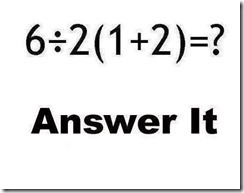## Wednesday, January 02, 2013

### Avoid Puzzles in Code

I keep seeing a lot of order of operations “puzzles” in my Facebook stream. The most recent one was 6/2*(1+2) = ? orA lot of people got it wrong. As you might expect the two answers I saw most were 1 and 9. Nine being the correct answer of course. I suspect that people remember the order of operations incompletely. That is to say that they know the order as Parentheses, Multiplication, Division, Addition and Subtraction. They add 1 to 2 getting three and then multiply 3 by 2 because “multiplication comes after parentheses and before division.” That is wrong though because multiplication and division are at the same level of order so the steps become left to right.
Six should be divided by two before that result is multiplied by 3. This gives the correct answer of nine. This could all be avoided by the use of one more set of parentheses: (6/2) * (1+2) is so much more clear and so much less ambiguous.
Now someone who is deeply immersed in math is not that likely to have a problem doing this correctly but most of us are not that immersed. In fact most of us really don’t want to have to think this deeply about the math. This is especially true in programming where we have so many other weird things to keep track of.
The rule of thumb for coding should be “don’t make the reader have to think anymore than absolutely necessary.” That means use parenthesis to avoid anyone having to think hard about order of operations. Some programmers like to show off how clever they are and do all sorts of tricky things. I think we need to instill the idea that smart programmers write code even a beginner can understand.

#### 1 comment:Anonymous said...

6/2(2+1) - parenthesis first - (2+1)=3; makes equation = 6/2(3)
6/2(3) - parenthesis first - 2(3) = 2 x 3 = 6
6/6 - division - 6 / 6 = 1
The equation equals 1.